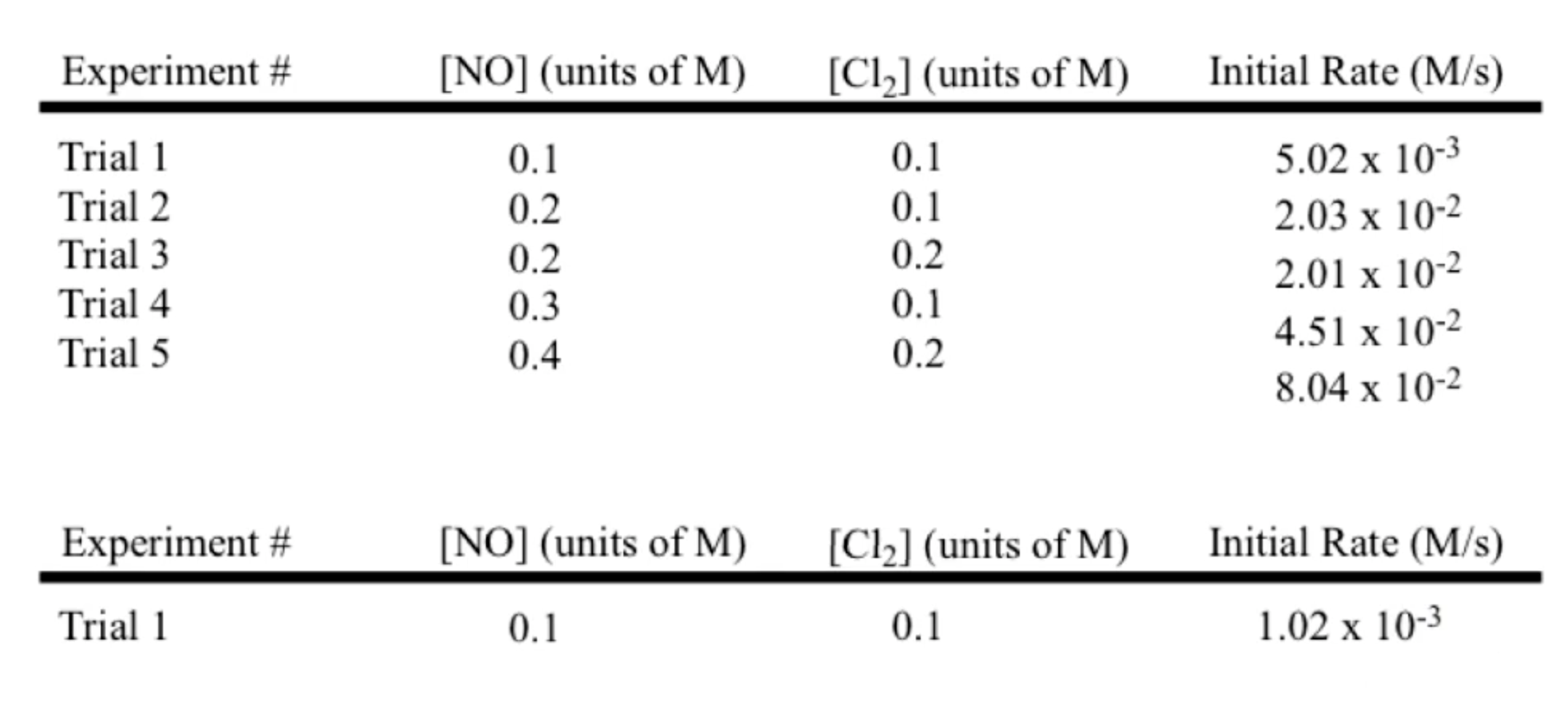# Problem: An experiment was run at a temperature of T= 496 K and the following initial rates were observed, after which the reaction was then run at a temperature of T = 298 K. Assuming the reaction orders have not changed with temperature, determine the new rate constant at this temperature, the activation energy (Ea), and pre-exponential/frequency factor, A.From the choices below, what is the calculated Ea?

92% (23 ratings)
###### Problem Details

An experiment was run at a temperature of T= 496 K and the following initial rates were observed, after which the reaction was then run at a temperature of T = 298 K.

Assuming the reaction orders have not changed with temperature, determine the new rate constant at this temperature, the activation energy (Ea), and pre-exponential/frequency factor, A.

From the choices below, what is the calculated Ea?